# Selina Solutions Concise Maths Class 7 Chapter 1: Integers Exercise 1C

Selina Solutions Concise Maths Class 7 Chapter 1 Integers Exercise 1C provides students with a clear idea of the removal of brackets and performing various operations on the integers. Numerous solved examples are present to help students understand the method of solving problems in a shorter duration. Students can boost their exam preparation by solving the textbook problems, using the solutions created by experts. These solutions contain explanations in a simple language, keeping in mind the intelligent coefficient of students. Students can access Selina Solutions Concise Maths Class 7 Chapter 1 Integers Exercise 1C free PDF, from the links which are available below.

## Selina Solutions Concise Maths Class 7 Chapter 1: Integers Exercise 1C Download PDF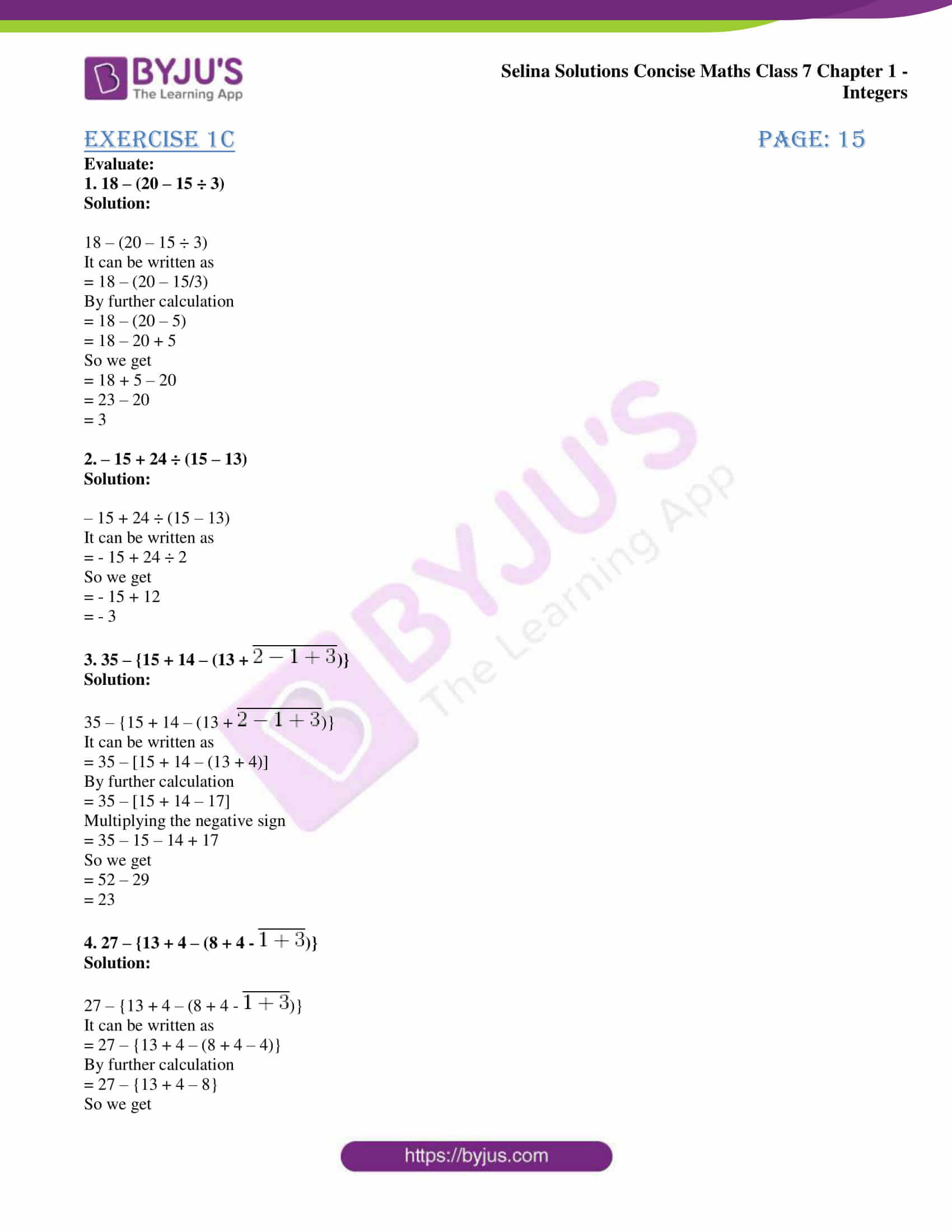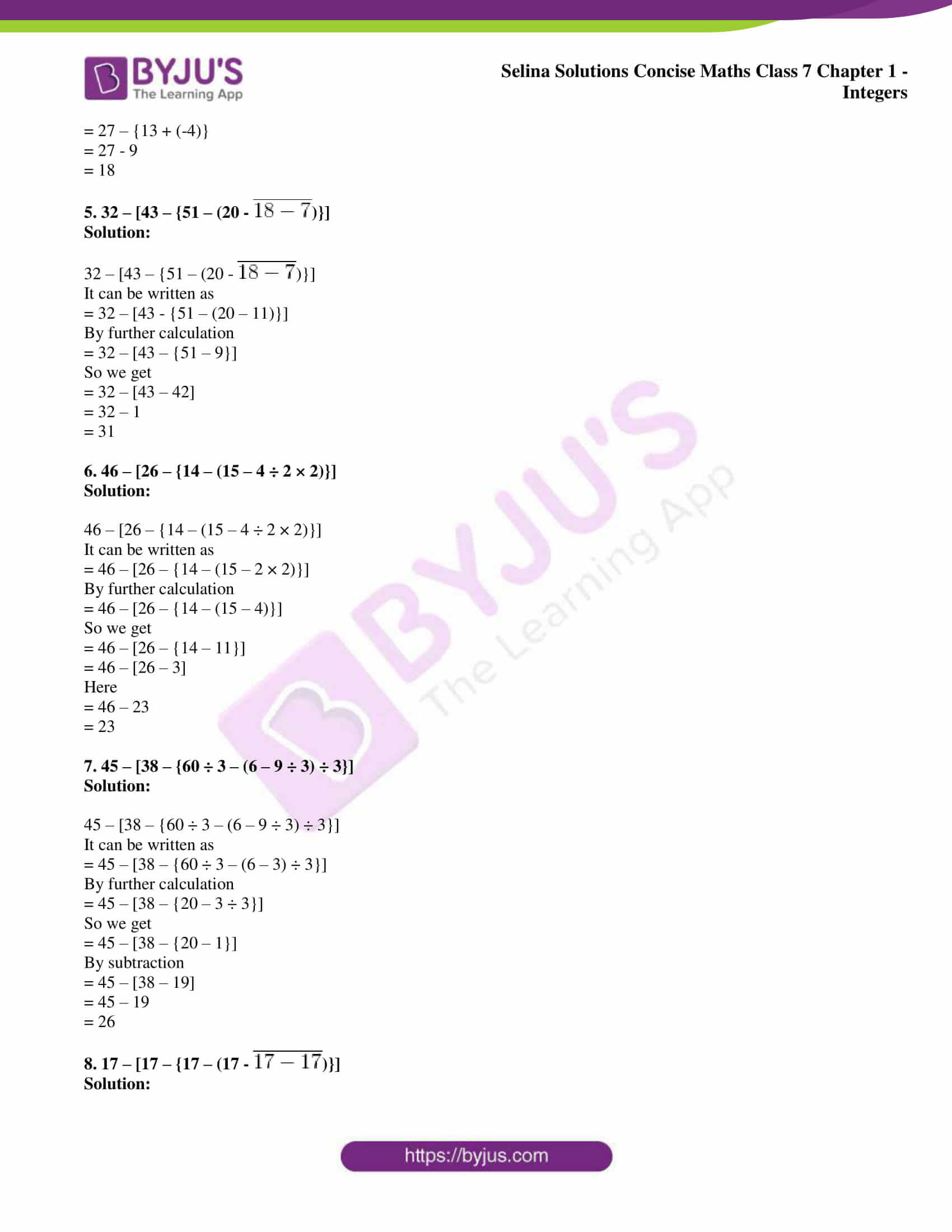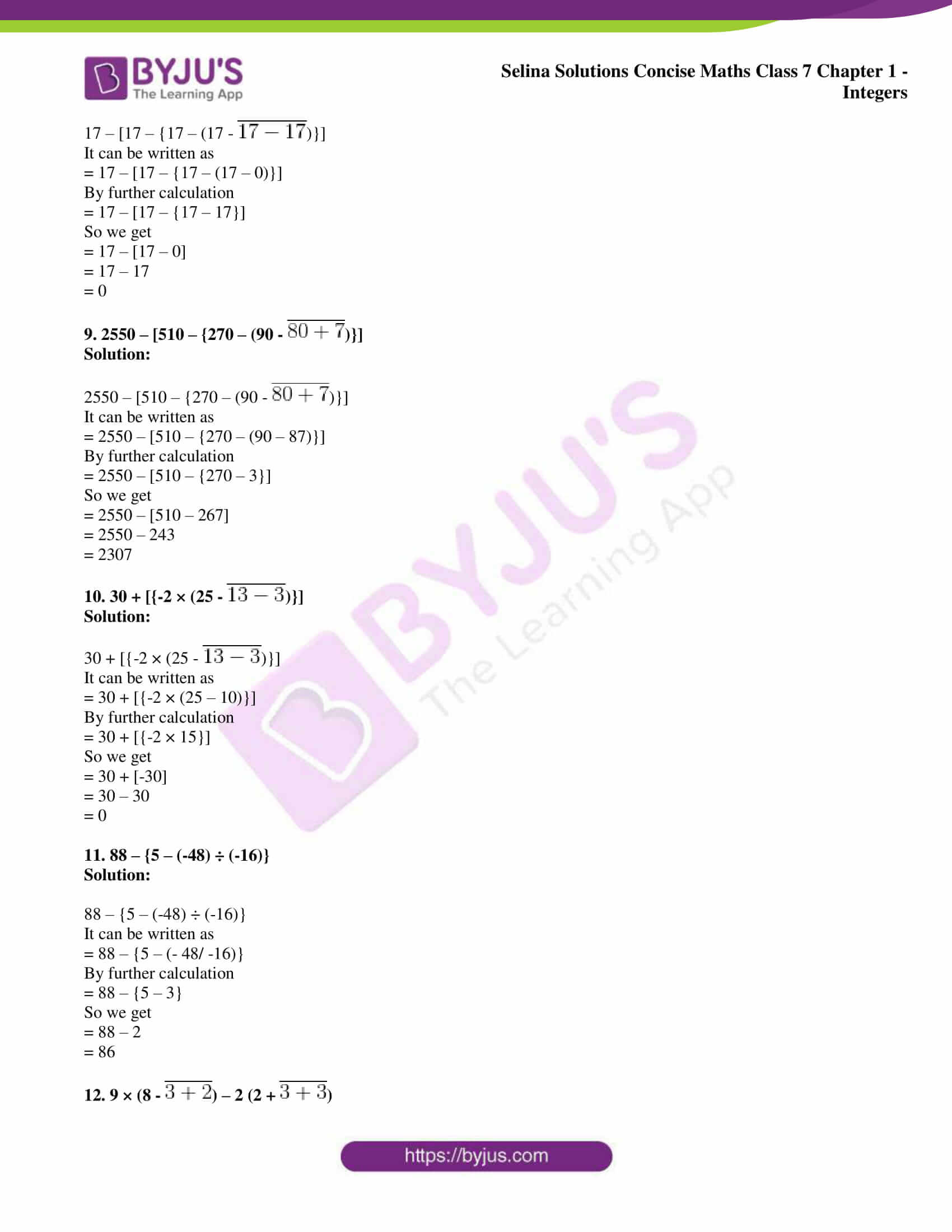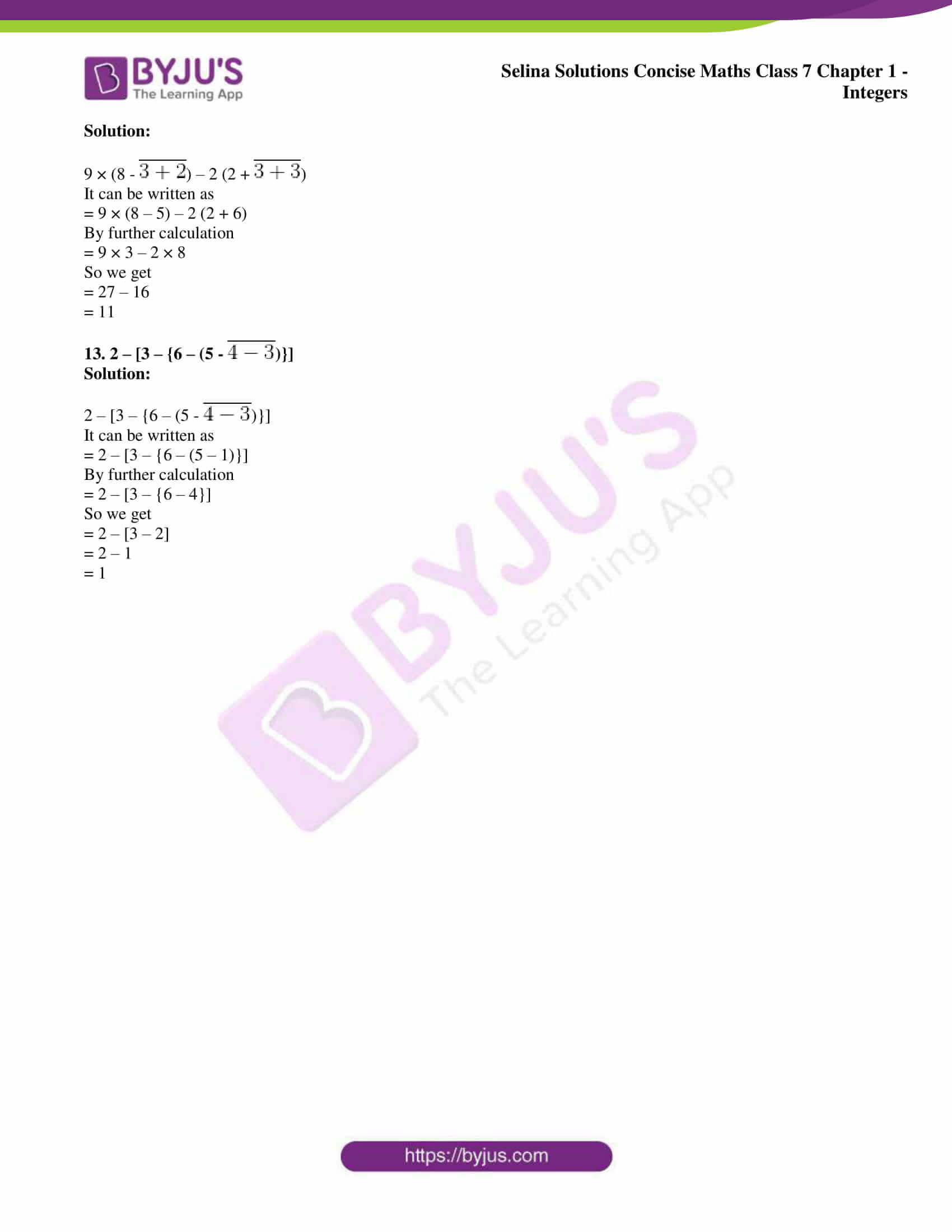### Access other exercises of Selina Solutions Concise Maths Class 7 Chapter 1: Integers

Exercise 1A Solutions

Exercise 1B Solutions

Exercise 1D Solutions

### Access Selina Solutions Concise Maths Class 7 Chapter 1: Integers Exercise 1C

Evaluate:

1. 18 – (20 – 15 ÷ 3)

Solution:

18 – (20 – 15 ÷ 3)

It can be written as

= 18 – (20 – 15/3)

By further calculation

= 18 – (20 – 5)

= 18 – 20 + 5

So we get

= 18 + 5 – 20

= 23 – 20

= 3

2. – 15 + 24 ÷ (15 – 13)

Solution:

– 15 + 24 ÷ (15 – 13)

It can be written as

= – 15 + 24 ÷ 2

So we get

= – 15 + 12

= – 3

3. 35 – {15 + 14 – (13 +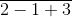)}

Solution:

35 – {15 + 14 – (13 +)}

It can be written as

= 35 – [15 + 14 – (13 + 4)]

By further calculation

= 35 – [15 + 14 – 17]

Multiplying the negative sign

= 35 – 15 – 14 + 17

So we get

= 52 – 29

= 23

4. 27 – {13 + 4 – (8 + 4 –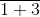)}

Solution:

27 – {13 + 4 – (8 + 4 –)}

It can be written as

= 27 – {13 + 4 – (8 + 4 – 4)}

By further calculation

= 27 – {13 + 4 – 8}

So we get

= 27 – {13 + (-4)}

= 27 – 9

= 18

5. 32 – [43 – {51 – (20 –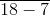)}]

Solution:

32 – [43 – {51 – (20 –)}]

It can be written as

= 32 – [43 – {51 – (20 – 11)}]

By further calculation

= 32 – [43 – {51 – 9}]

So we get

= 32 – [43 – 42]

= 32 – 1

= 31

6. 46 – [26 – {14 – (15 – 4 ÷ 2 × 2)}]

Solution:

46 – [26 – {14 – (15 – 4 ÷ 2 × 2)}]

It can be written as

= 46 – [26 – {14 – (15 – 2 × 2)}]

By further calculation

= 46 – [26 – {14 – (15 – 4)}]

So we get

= 46 – [26 – {14 – 11}]

= 46 – [26 – 3]

Here

= 46 – 23

= 23

7. 45 – [38 – {60 ÷ 3 – (6 – 9 ÷ 3) ÷ 3}]

Solution:

45 – [38 – {60 ÷ 3 – (6 – 9 ÷ 3) ÷ 3}]

It can be written as

= 45 – [38 – {60 ÷ 3 – (6 – 3) ÷ 3}]

By further calculation

= 45 – [38 – {20 – 3 ÷ 3}]

So we get

= 45 – [38 – {20 – 1}]

By subtraction

= 45 – [38 – 19]

= 45 – 19

= 26

8. 17 – [17 – {17 – (17 –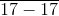)}]

Solution:

17 – [17 – {17 – (17 –)}]

It can be written as

= 17 – [17 – {17 – (17 – 0)}]

By further calculation

= 17 – [17 – {17 – 17}]

So we get

= 17 – [17 – 0]

= 17 – 17

= 0

9. 2550 – [510 – {270 – (90 –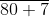)}]

Solution:

2550 – [510 – {270 – (90 –)}]

It can be written as

= 2550 – [510 – {270 – (90 – 87)}]

By further calculation

= 2550 – [510 – {270 – 3}]

So we get

= 2550 – [510 – 267]

= 2550 – 243

= 2307

10. 30 + [{-2 × (25 –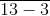)}]

Solution:

30 + [{-2 × (25 –)}]

It can be written as

= 30 + [{-2 × (25 – 10)}]

By further calculation

= 30 + [{-2 × 15}]

So we get

= 30 + [-30]

= 30 – 30

= 0

11. 88 – {5 – (-48) ÷ (-16)}

Solution:

88 – {5 – (-48) ÷ (-16)}

It can be written as

= 88 – {5 – (- 48/ -16)}

By further calculation

= 88 – {5 – 3}

So we get

= 88 – 2

= 86

12. 9 × (8 –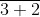) – 2 (2 +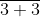)

Solution:

9 × (8 –) – 2 (2 +)

It can be written as

= 9 × (8 – 5) – 2 (2 + 6)

By further calculation

= 9 × 3 – 2 × 8

So we get

= 27 – 16

= 11

13. 2 – [3 – {6 – (5 –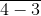)}]

Solution:

2 – [3 – {6 – (5 –)}]

It can be written as

= 2 – [3 – {6 – (5 – 1)}]

By further calculation

= 2 – [3 – {6 – 4}]

So we get

= 2 – [3 – 2]

= 2 – 1

= 1